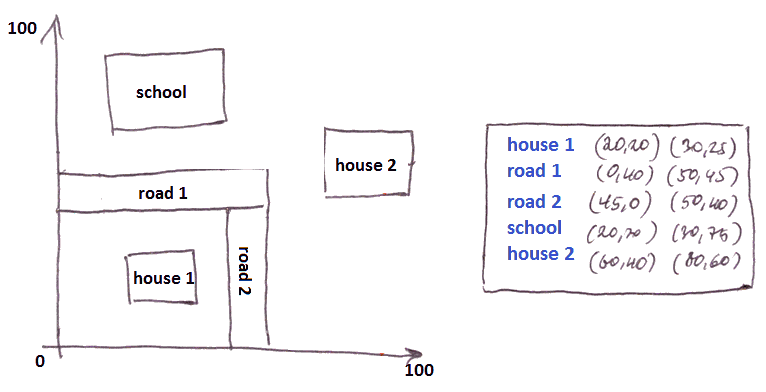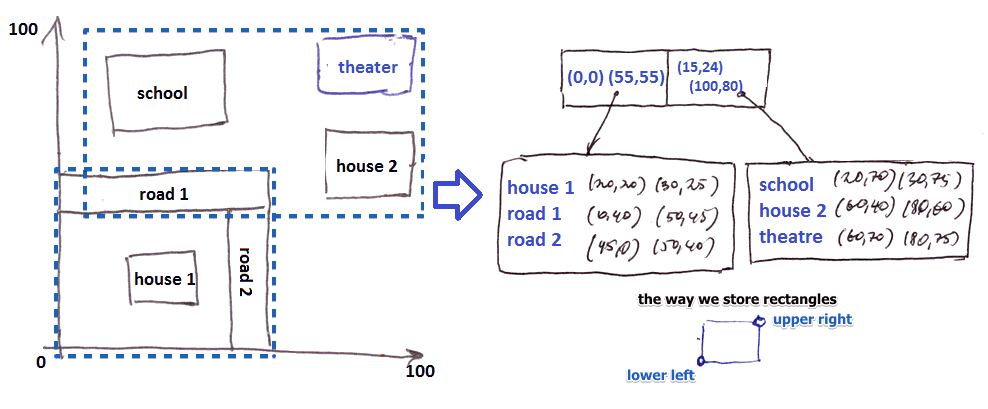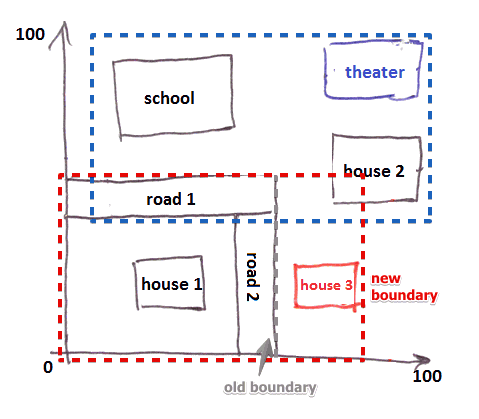# ML Wiki

## R-Tree

• Generalization of a B-Tree to multidimensional space
• Indexes regions

### B-Tree

In a B-Tree we can view a node as a line (1-dimensional space)

•• and it divides a line into segments

### R-Tree

Same, but for 2D and more

• we divide data into data regions
• interior nodes of an R-Tree correspond to interior region
• not data region as in B-Tree, but just a region
• A region can be of any shape, but usually it's a rectangle or other simple shape
• A node has subregions - its children
• subregions are allowed to overlap
• but it's usually better to keep the overlap small

## Example

Suppose we have a region

• it fits in one block
•• but we insert an new object - and it no longer fits
• need to split the block into two regions
•• note that (a) the blocks overlap and (b) how we represent these blocks in out database
• when we insert next time, a new object can be added to an existent block
•• note that we have to adjust regions boundaries to include the new object

## Operations

### Lookup

specify a point $P$ and ask what regions $P$ lies in (where-am-I query)

• find which children correspond to interior regions that contain $P$
• if there are no such regions - we're done ($P$ doesn't belong to any region)
• if there are more than 1 region - apply recursively to each
• when we reach the leaf regions - we find the actual data regions

### Insert

• start at root and try to find a region where $R$ fits
• if found: go inside and repeat
• if not: need to expand an existing region
• we want to expand as little as possible
• so we find the one that gives the smallest expansion
• when we reach a leaf, we insert $R$
• if there's no room - we split it
• remember that we want regions to be as small as possible
• so we find the split that gives us that
• after that we insert the new subregion to the leaf's parent
• essentially the same procedure as for B-Tree

## Summary

Good for:

• Where-am-I (point) queries
• Finding intersecting regions (e.g. when a user selects an area on map)
• Partial Range queries
• Range queries
• nearest neighbor

Also

• Always balanced
• often used in practice